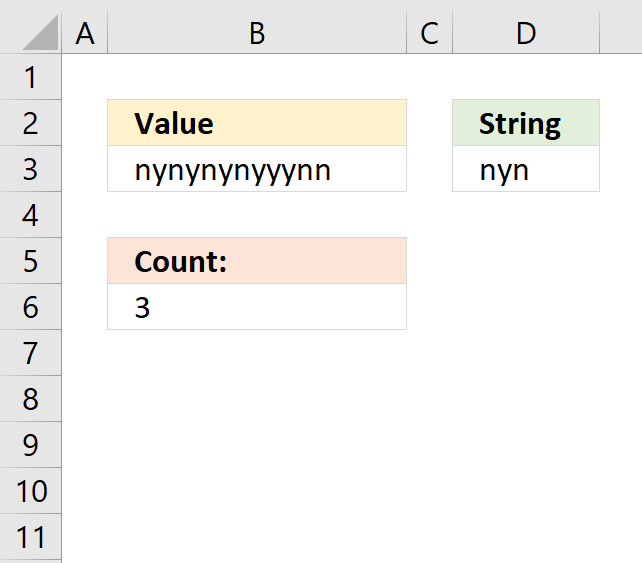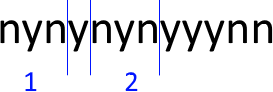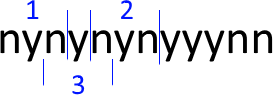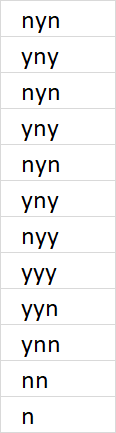Author: Oscar Cronquist Article last updated on October 31, 2018The formula in cell B6 counts how many times the string (D3) is found in a cell value (B3) even if it overlaps another match.

Formula in cell B6:

=SUMPRODUCT(SIGN(MID(B3, ROW(A1:INDEX(A1:A1000, LEN(B3))), LEN(D3))=D3))

A regular count would result in 2 matches, see picture below.
You can find the formula here: Count specific text string in a cellA count where overlapping is allowed returns 3 matches and this is what is demonstrated in this article.### Explaining formula in cell B6

#### Step 1 - Build an array from 1 to the number of characters in the cell value

The LEN function counts the number of characters in cell B3.

LEN(B3) returns 13.

The INDEX function returns a cell reference based on a row number.

ROW(A1:INDEX(A1:A1000, LEN(B3)))

becomes

ROW(A1:INDEX(A1:A1000, 13))

becomes

ROW(A1:A13) and returns {1; 2; 3; 4; 5; 6; 7; 8; 9; 10; 11; 12; 13}

The ROW function returns the row number of a cell. If a cell range is used the ROW function returns an array of row numbers.

#### Step 2 - Extract all possible substrings from cell value

MID(B3, ROW(A1:INDEX(A1:A1000, LEN(B3))), LEN(D3))

becomes

MID(B3, {1; 2; 3; 4; 5; 6; 7; 8; 9; 10; 11; 12; 13}, LEN(D3))

becomes

MID("nynynynyyynn", {1; 2; 3; 4; 5; 6; 7; 8; 9; 10; 11; 12; 13}, 3)

and returns the following array:#### Step 3 - Check if substring is equal to search string

MID(B3, ROW(A1:INDEX(A1:A1000, LEN(B3))), LEN(D3))=D3

becomes

{"nyn"; "yny"; "nyn"; "yny"; "nyn"; "yny"; "nyy"; "yyy"; "yyn"; "ynn"; "nn "; "n "; " "}=D3

and returns {TRUE; FALSE; TRUE; FALSE; TRUE; FALSE; FALSE; FALSE; FALSE; FALSE; FALSE; FALSE; FALSE}.

#### Step 4 - Convert boolean values to the corresponding number

The SUMPRODUCT function can't handle boolean values so the SIGN function converts them into numbers. TRUE = 1 and FALSE = 0.

SIGN(MID(B3, ROW(A1:INDEX(A1:A1000, LEN(B3))), LEN(D3))=D3)

becomes SIGN({TRUE; FALSE; TRUE; FALSE; TRUE; FALSE; FALSE; FALSE; FALSE; FALSE; FALSE; FALSE; FALSE})

and returns {1; 0; 1; 0; 1; 0; 0; 0; 0; 0; 0; 0; 0}.

#### Step 5 - Count values in array

SUMPRODUCT(SIGN(MID(B3, ROW(A1:INDEX(A1:A1000, LEN(B3))), LEN(D3))=D3))

becomes

SUMPRODUCT({1; 0; 1; 0; 1; 0; 0; 0; 0; 0; 0; 0; 0})

and returns 3 in cell B6. 1 + 0 + 1 + 0 + 1 + 0 + 0 + 0 + 0 + 0 + 0 + 0 + 0 = 3.

### Download Excel *.xlsx file

Count overlapping text string in a cell.xlsx